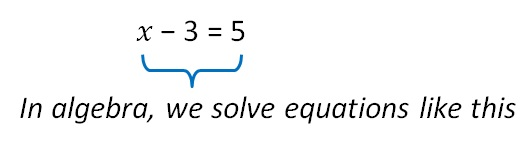Chapter 11 Class 6 Algebra
Concept wise

Suppose we want to solve

⎕ − 3 = 5

We have to find the missing number

In algebra,

We don’t write boxes

It has a variable − x

We write letters, like 𝑥It has a variable − x

But, why write 𝑥? Why not boxes ?

• Writing x is easier to write than ⎕
• if there are two boxes, now do you tell which box is which ?

So let’s start ?

Learn in your speed, with individual attention - Teachoo Maths 1-on-1 Class